# Test: Array & Linked List

## 20 Questions MCQ Test GATE Computer Science Engineering(CSE) 2023 Mock Test Series | Test: Array & Linked List

Description
Attempt Test: Array & Linked List | 20 questions in 60 minutes | Mock test for Computer Science Engineering (CSE) preparation | Free important questions MCQ to study GATE Computer Science Engineering(CSE) 2023 Mock Test Series for Computer Science Engineering (CSE) Exam | Download free PDF with solutions
QUESTION: 1

### In a compact single dimensional array representation for lower triangular matrices (i.e all the elements above the diagonal are zero) of size n X n, non-zero elements, (i.e elements of lower triangle) of each row are stored one after another, starting from the first row, the index of the (i,j)th  element of the lower triangular matrix in this new representation is:

Solution:

j+(sum of natural number till i-1) because if you form a lower triangular matrix it contains elements in rows 1,2,3,...
So C is the correct answer.
PS: Though not mentioned in question, from options it is clear that array index starts from 1 and not 0.

QUESTION: 2

### Let A be a two dimensional array declared as follows: A: array [1 …. 10] [1 ….. 15] of integer; Assuming that each integer takes one memory location, the array is stored in row-major order and the first element of the array is stored at location 100, what is the address of the element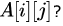Solution:

A [ LB1..............UB1,LB2.................UB2 ]

C = size of each element.

Row major order.

Loc(a[i][j]) = BA + [ (i-LB1) (UB2 - LB2 + 1) + (j - LB2) ] * C.

Column Major order

Loc(a[i][j]) = BA + [ (j-LB2) (UB1 - LB1 + 1) + (i - LB1) ] * C.

substituting the values. answer is A.

QUESTION: 3

### An n * n array ν is defined as follows :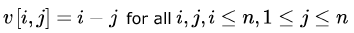The sum of the elements of the array v is

Solution:

The sum of the ith row and ith column is 0 as shown below. Since, the numbers of rows = no. of columns, the total sum will be 0.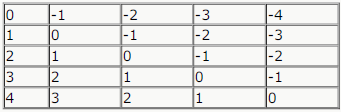QUESTION: 4

A program P reads in 500 integers in the range [0, 100] representing the scores of 500 students. It then prints the frequency of each score above 50. What would be the best way for P to store the frequencies?

Solution:

as we our area of interest is only the 50 numbers so take An array of 50 numbers where A corresponds to 51...A
corresponds to 100 then after reading an input just increment the counter in correct position as said above

QUESTION: 5

The procedure given below is required to find and replace certain characters inside an input character string supplied in array A. The characters to be replaced are supplied in array oldc, while their respective replacement characters are supplied in array newc. Array A has a fixed length of five characters, while arrays oldc and newc contain three characters each.
However, the procedure is flawed.

void find_and_replace (char *A, char *oldc, char *newc) {

for (int i=0; i<5; i++)

for (int j=0; j<3; j++)

if (A[i] == oldc[j])

A[i] = newc[j];

}

The procedure is tested with the following four test cases.

(1) oldc = “abc”, newc = “dab”

(2) oldc = “cde”, newc = “bcd”

(3) oldc = “bca”, newc = “cda”

(4) oldc = “abc”, newc = “bac”

The tester now tests the program on all input strings of length five consisting of characters ‘a’, ‘b’, ‘c’, ‘d’ and ‘e’ with duplicates allowed. If the tester carries out this testing with the four test cases given above, how many test cases will be able to capture the flaw?

Solution: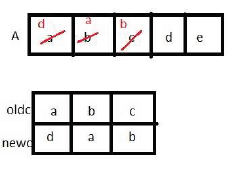Here when the element of array A and oldc match , we replace that array element of A with array element of newc . For every element of A array update occurs maximum one time.

Similarly for (2) array element of A has updated with array element of newc less than or equal to one time,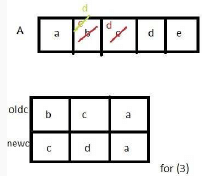Now, for (3) when i=0 , value of A match with oldc i.e.'a' , and replace with newc i.e. also 'a'. So, no changes
when i=1 value of array A='b'
match with oldc='b' and replace with newc='c'.
Now, A='c' which equal with next element of oldc='c'.
So, replace again with newc='d'.
Now, we can say here in array A value replace with newc value , and that newc value replace with next newc
value.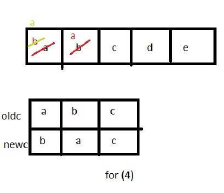Similarly for (4) here 2 times replacement for A with element newc and newc
Updating of newc value with another newc value is calling flaw here
So Ans B

QUESTION: 6

The procedure given below is required to find and replace certain characters inside an input character string supplied in array A. The characters to be replaced are supplied in array oldc, while their respective replacement characters are supplied in array newc. Array A has a fixed length of five characters, while arrays oldc and newc contain three characters each.
However, the procedure is flawed.

void find_and_replace (char *A, char *oldc, char *newc) {

for (int i=0; i<5; i++)

for (int j=0; j<3; j++)

if (A[i] == oldc[j])

A[i] = newc[j];

}

The procedure is tested with the following four test cases.

(1) oldc = “abc”, newc = “dab”

(2) oldc = “cde”, newc = “bcd”

(3) oldc = “bca”, newc = “cda”

(4) oldc = “abc”, newc = “bac”

If array A is made to hold the string “abcde”, which of the above four test cases will be successful in exposing the flaw in this procedure?

Solution: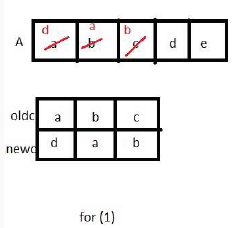Here when the element of array A and oldc match , we replace that array element of A with array element of newc . For every element of A array update occurs maximum one time.

Similarly for (2) array element of A has updated with array element of newc less than or equal to one time,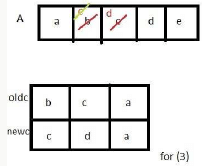Now, for (3) when i=0 , value of A match with oldc i.e.'a' , and replace with newc i.e. also 'a'. So, no changes

when i=1 value of array A='b'

match with oldc='b' and replace with newc='c'.

Now, A='c' which equal with next element of oldc='c'.

So, replace again with newc='d'.

Now, we can say here in array A value replace with newc value , and that newc value replace with next newc value.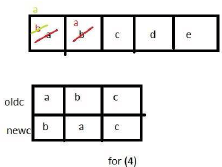Similarly for (4) here 2 times replacement for A with element newc and newc

Updating of newc value with another newc value is calling flaw here

QUESTION: 7

Consider the C function given below. Assume that the array list A contains n (> 0) elements, sorted in ascending order.

int ProcessArray(int *listA, int x, int n)

{
int i, j, k;

i = 0;     j = n-1;

do {

k = (i+j)/2;

if (x <= listA[k]) j = k-1;

if (listA[k] <= x) i = k+1;

}

while (i <= j);

if (listA[k] == x) return(k);

else return -1;

}

Which one of the following statements about the function Process Array is CORRECT?

Solution:

By the logic of the algorithm it is clear that it is an attempted implementation of Binary Search. So option C is clearly eliminated. Let us now check for options A and D. A good way to do this is to create small dummy examples (arrays) and implement the algorithm as it is. One may make any array of choice. Running iterations of the algorithm would indicate that the loop exits when the x is not present. So option A is wrong. Also, when x is present, the correct index is indeed returned. D is also wrong. Correct answer is B. It is a correct implementation of Binary Search.

*Answer can only contain numeric values
QUESTION: 8

A Young tableau is a 2D array of integers increasing from left to right and from top to bottom. Any unfilled entries are marked with ∞, and hence there cannot be any entry to the right of, or below a ∞. The following Young tableau consists of unique entries.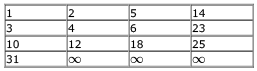When an element is removed from a Young tableau, other elements should be moved into its place so that the resulting table is still a Young tableau (unfilled entries may be filled with a ∞). The minimum number of entries (other than 1) to be shifted, to remove 1 from the given Young tableau is _____.

Solution:

1.We first need to shift 2 in place of 1 keeping 5 AND 14 intact as it isn't mentioned in the question that the entire row
elements move.
2. 4 is shifted up,next to 2(keeping 12 and infinity intact in column 2).
3. Now in second row 6 is shifted left.
4.18 shifts up to the second row
5. And finally 25 is shifted left to the third column.
So this takes 5 moves and still maintains the tableau property. Also infinity is placed to the right of 25 and below 23(unfilled entries to be filled with ∞). The final table would look as follows.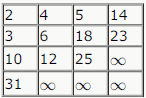QUESTION: 9

Consider an array A [1......n]. It consists of a permutation of numbers 1....n. Now compute another array B [1.....n] as follows: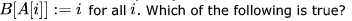Solution:

B is a permutation of array A

Infact, B gives the reverse index of all the elements of array A. Since the array A contains numbers [1..n] mapped to the locations [1...n] and A is a permutation of the numbers [1...n], the array B will also be a permutation of the numbers [1...n]. For example:

.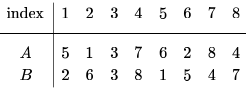To see that option c is incorrect, let array C be the array attained from doing the same transformation twice, that is,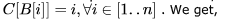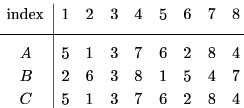We can see that C = A, which makes option c incorrect.

QUESTION: 10

In a circular linked list oraganisation, insertion of a record involves modification of

Solution:

suppose we have to insert node p after node q then
p->next=q->next
q->next=p
so two pointers
b)

QUESTION: 11

Linked lists are not suitable data structures for which one of the following problems?

Solution:

Insertion sort:->>No need to swap here just find appropriate place and join the link
Polynomial manipulation:->> Linked List is natural soln for polynomial manipulation .http://iiith.vlab.co.in/?
sub=21&brch=46&sim=490&cnt=697
Radix sort:->here we are putting digits according to same position(unit,tens) into buckets;
which can be effectively handle by linked list.
Not Suitable for
Binary search:-> Bcz finding mid element itself takes O(n) time.
So Option B is ans.

QUESTION: 12

Which of the following statements is true?

I. As the number of entries in a hash table increases, the number of collisions increases.

II. Recursive programs are efficient

III. The worst case complexity for Quicksort is O (n2)

IV. Binary search using a linear linked list is efficient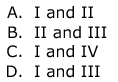Solution:

Binary search using linked list is not efficient as it will not give O(logn), because we will not be able to find the mid in constant time. Finding mid in linked list takes O(n) time.

Recursive programs are not efficient because they take a lot of space, Recursive methods will often throw
Stack Overflow  Exception while processing big sets. moreover it has its own advantages too.

QUESTION: 13

The concatenation of two lists is to be performed on O (1) time. Which of the following implementations of a list should be used?

Solution:

A) & B) Here it is not possble to do it in O(1) , unless we have pointer to end of one list. As we have not given that pointer, A & B are not option.
D) It is not possible to do here in O(1), because we will need to allocate memory for bigger array to hold both list & Copy it.
C) It is possible in O(1) as we can break list at any location & connect it anywhere. We don't need to traverse to end of anything here !

QUESTION: 14

In the worst case, the number of comparisons needed to search a single linked list of length n for a given element is

Solution:

A & C is not correct as we can not do binary search in Linked list.
B seems like average case, be we are asked for worst case.
Worst case is we do not find the element in list. We might end up searching entire list & comparing with each element. So

QUESTION: 15

Consider the function f defined below.

struct item {

int data;

struct item * next;

};

int f(struct item *p) {

return ((p == NULL) || (p->next == NULL)||

((p->data <= p ->next -> data) &&

f(p->next)));

}

For a given linked list p, the function  f returns 1 if and only if

Solution:

It returns 1 if and only if the linked list is sorted in non-decreasing order- B option.
It returns 1 if the list is empty or has just 1 element also, but if and only if makes A option false.

QUESTION: 16

A circularly linked list is used to represent a Queue. A single variable p is used to access the Queue. To which node should point such that both the operations enqueue and dequeue can be performed in constant time?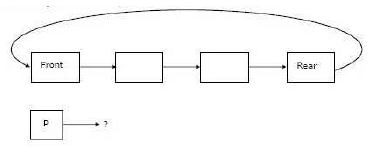Solution:

The pointer points to the Rear node.

EnQueue: Insert newNode after Rear, and make Rear point to the newly inserted node:

//struct node *newNode;
newNode->next = rear->next;
rear->next = newNode;
rear=newNode;

DeQueue: Delete the Front node, and make the second node the front node.

//rear->next points to the front node.
//front->next points to the second node.
struct node* front;
front = rear->next;
rear->next = front->next;
free(front);

QUESTION: 17

Suppose each set is represented as a linked list with elements in arbitrary order. Which of the operations among  Union, intersection,membership,cardinality will be the slowest?

Solution:

membership is linear search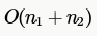cardinality is linear -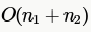for union we need to ensure no duplicate elements should be present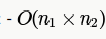for each element we need to check if
that element exists in other set for intersection also for every element in set1 we need to scan set2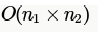QUESTION: 18

Let P be a singly linked list. Let Q be the pointer to an intermediate node x in the list. What is the worst-case time complexity of the best-known algorithm to delete the node x from the list ?

Solution:

simple solution is to traverse the linked list until you find the node you want to delete. But this solution requires pointer to the head node which contradicts the problem statement.

Time complexity of this approach is O(1)

QUESTION: 19

The following C function takes a singly-linked list of integers as a parameter and rearranges the elements of the list. The list is represented as pointer to a structure. The function is called with the list containing the integers 1, 2, 3, 4, 5, 6, 7 in the given order. What will be the contents of the list after the function completes execution?

struct node {int value; struct node *next;);

void rearrange (struct node *list) {

struct node *p, *q;

int temp;

if (!list || !list -> next) return;

p = list; q = list -> next;

while (q) {

temp = p -> value;

p -> value = q -> value;

q -> value = temp;

p = q -> next;

q = p ? p -> next : 0;

}

}

Solution:

i think it's B) 2 1 4 3 6 5 7:
as,
p and q are swapping each other.where q is p->next all the time.

QUESTION: 20

The following C function takes a single-linked list of integers as a parameter and rearranges the elements of the list. The function is called with the list containing the integers 1, 2, 3, 4, 5, 6, 7 in the given order. What will be the contents of the list after function completes execution?

struct node {

int value;

struct node *next;

};

void rearrange(struct node *list) {

struct node *p, *q;

int temp;

if (!list || !list -> next) return;

p = list; q = list -> next;

while(q) {

temp = p -> value; p->value =  q -> value;

q->value = temp; p = q ->next;

q = p? p ->next : 0;

}
}

Solution:

The loop is interchanging the adjacent elements of the list. But after each interchange, next interchange starts from the
unchanged elements only (due to p = q -> next;).
1st iteration 1 2 3 4 5 6 7
=> 2 1 3 4 5 6 7
2nd iteration 2 1 4 3 5 6 7
3rd iteration 2 1 4 3 6 5 7
p pointing to null q pointing to 7, as p is false hence q=p? p->next:0; will return q=0 ending the loopUse Code STAYHOME200 and get INR 200 additional OFF Use Coupon Code